# How to Calculate and Solve for Partial Dislocation Separation | Fracture Mechanics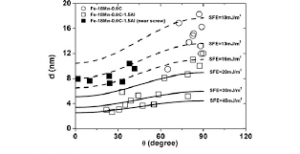The image above represents partial dislocation separation.

To compute for partial dislocation separation, four essential parameters are needed and these parameters are Shear Modulus (G), Burger Vector (b2), Burger Vector (b3and Stacking Fault Energy (γ).

The formula for calculating partial dislocation separation:

d = Gb2b3 / 2πγ

Where:

d = Partial Dislocation Separation
G = Shear Modulus
b2 and b3 = Burger Vectors
γ = Stacking Fault Energy

Let’s solve an example;
Find the partial dislocation separation when the shear modulus is 4. the burger vectors is 8 and 10, the stacking fault energy is 14.

This implies that;

G = Shear Modulus = 4
b2 and b3 = Burger Vectors = 8 and 10
γ = Stacking Fault Energy = 14

d = Gb2b3 / 2πγ
d = (4)(8)(10) / 2π(14)
d = 320 / 87.96
d = 3.64

Therefore, the partial dislocation separation is 3.64 m.

Nickzom Calculator – The Calculator Encyclopedia is capable of calculating the partial dislocation separation.

To get the answer and workings of the partial dislocation separation using the Nickzom Calculator – The Calculator Encyclopedia. First, you need to obtain the app.

You can get this app via any of these means:

To get access to the professional version via web, you need to register and subscribe for NGN 2,000 per annum to have utter access to all functionalities.
You can also try the demo version via https://www.nickzom.org/calculator

Android (Paid) – https://play.google.com/store/apps/details?id=org.nickzom.nickzomcalculator
Android (Free) – https://play.google.com/store/apps/details?id=com.nickzom.nickzomcalculator
Apple (Paid) – https://itunes.apple.com/us/app/nickzom-calculator/id1331162702?mt=8
Once, you have obtained the calculator encyclopedia app, proceed to the Calculator Map, then click on Materials and Metallurgical under Engineering.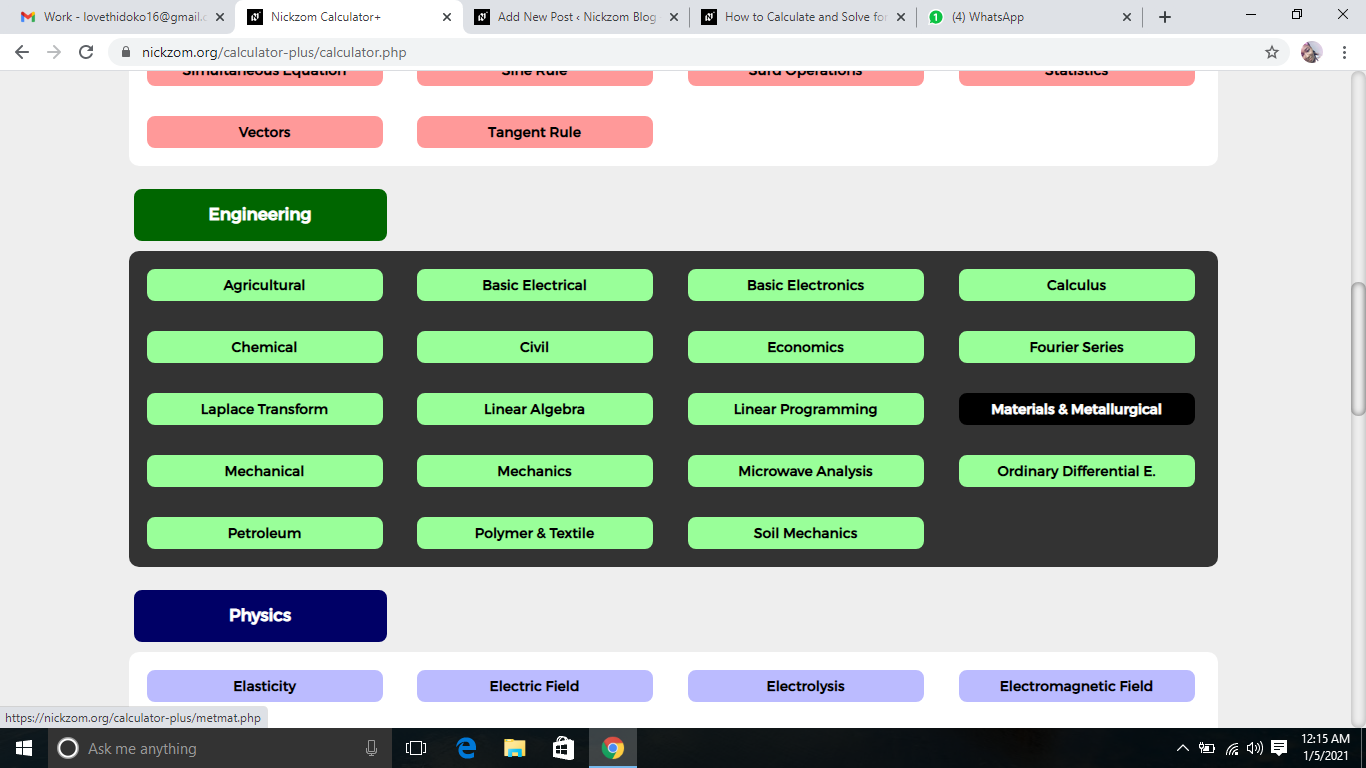Now, Click on Fracture Mechanics under Materials and MetallurgicalNow, Click on Partial Dislocation Separation under Fracture Mechanics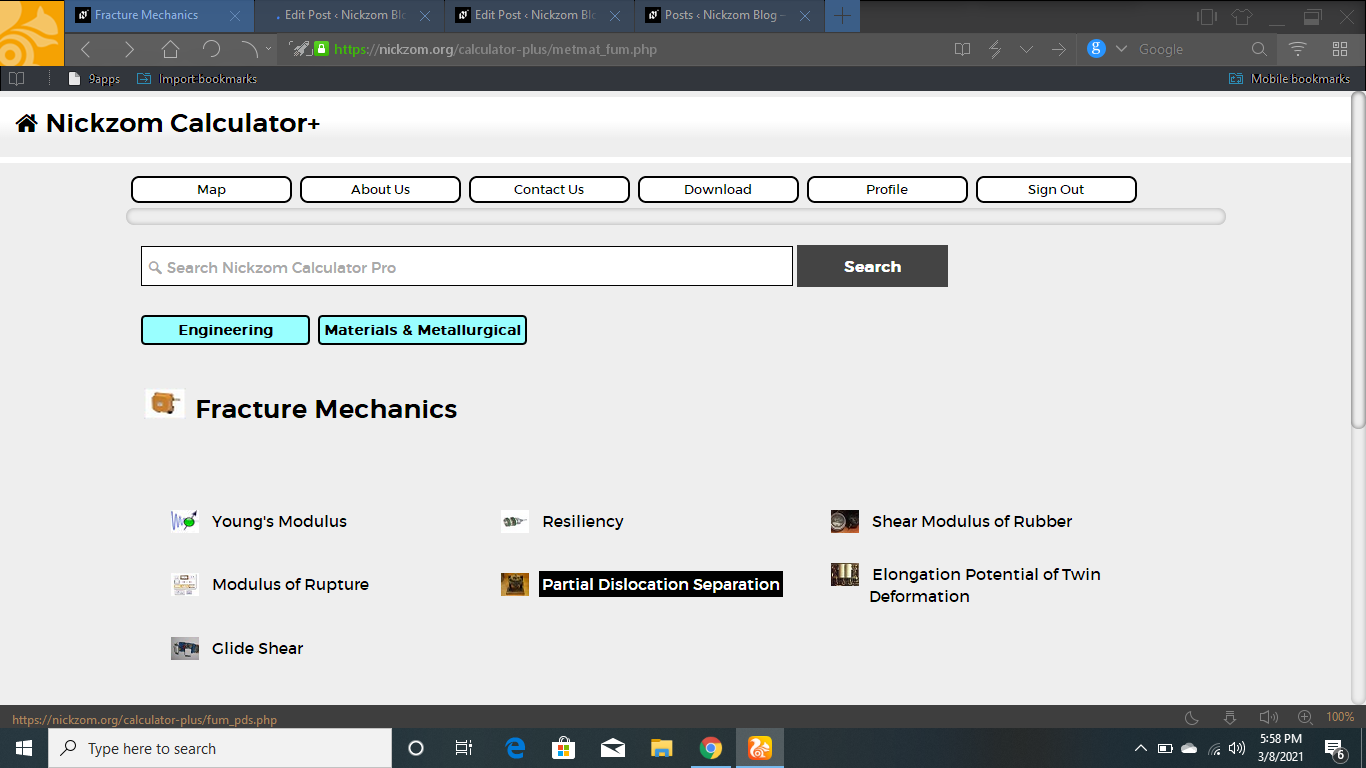The screenshot below displays the page or activity to enter your values, to get the answer for the partial dislocation separation according to the respective parameters which is the Shear Modulus (G), Burger Vector (b2), Burger Vector (b3and Stacking Fault Energy (γ).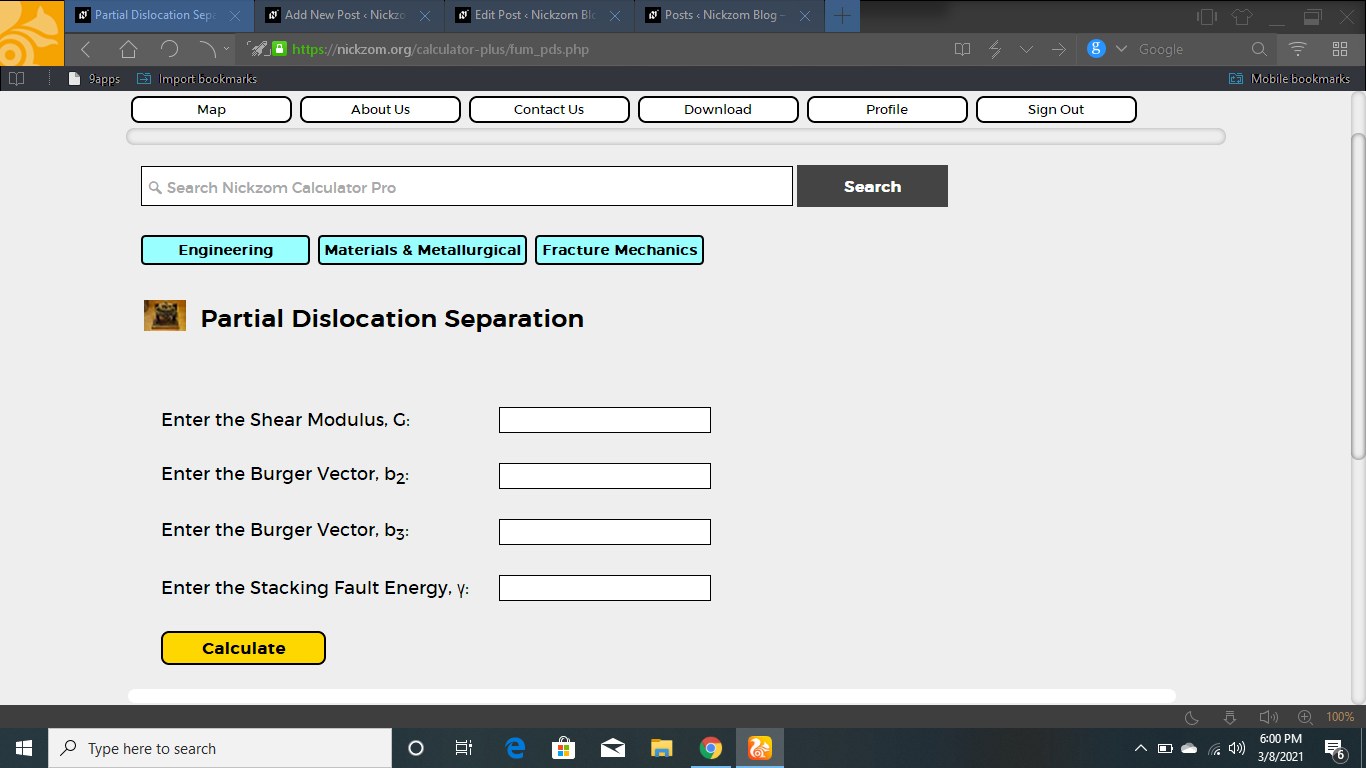Now, enter the value appropriately and accordingly for the parameter as required by the Shear Modulus (G) is 4, Burger Vector (b2) is 8, Burger Vector (b3) is 10 and Stacking Fault Energy (γ) is 14.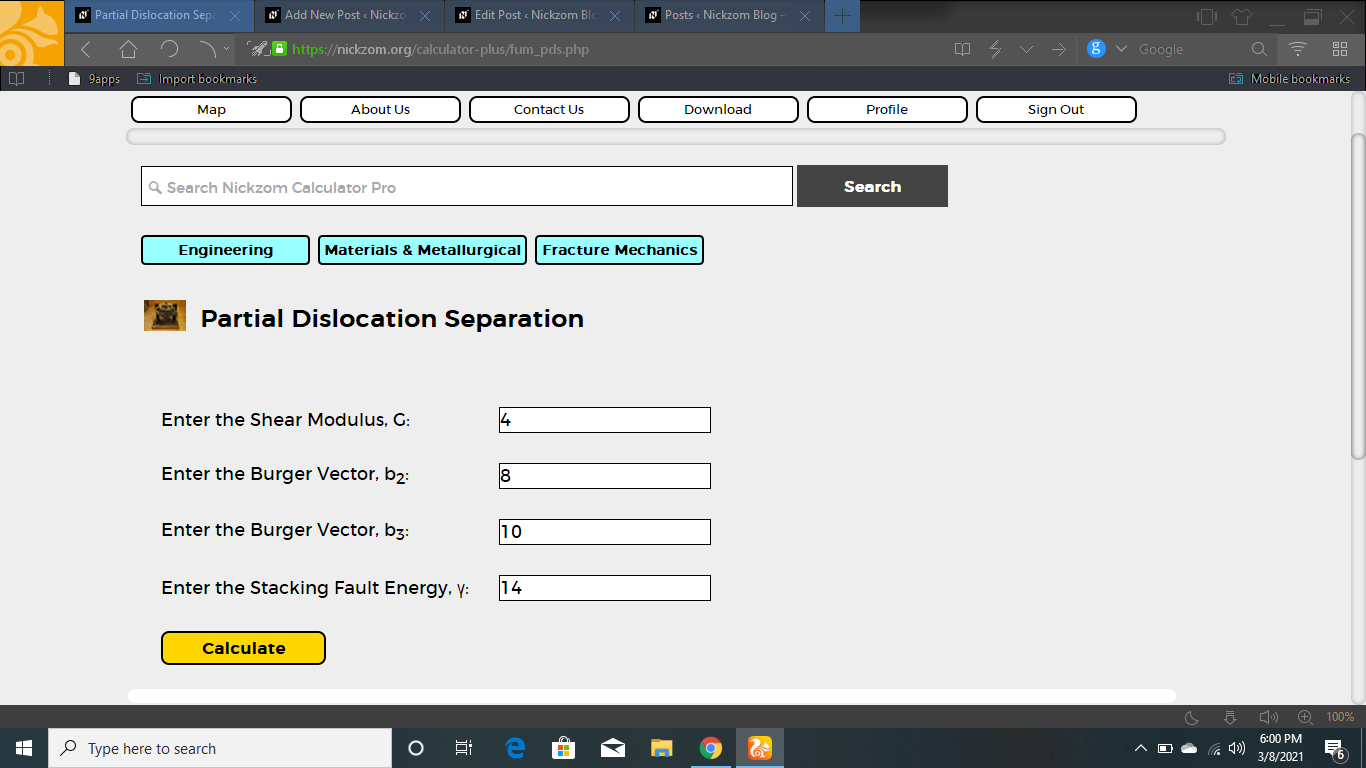Finally, Click on Calculate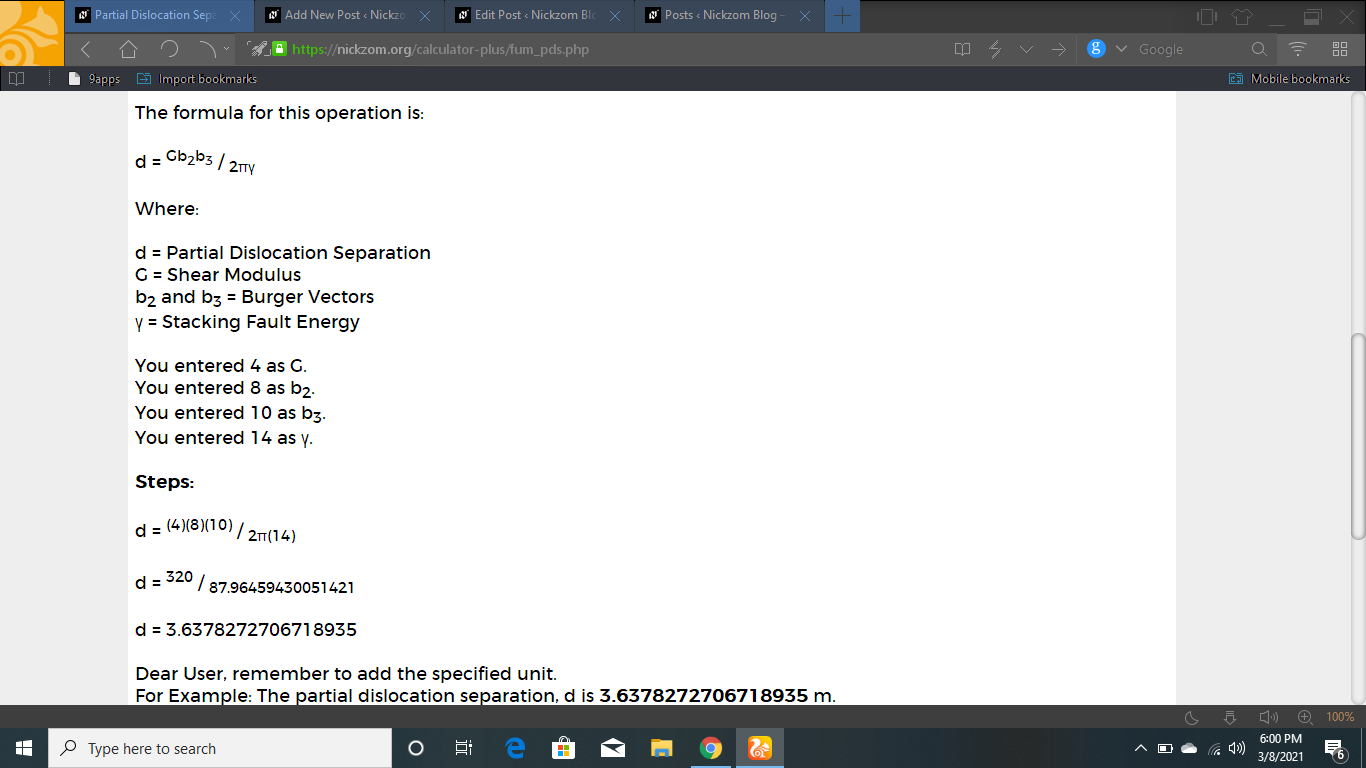As you can see from the screenshot above, Nickzom Calculator– The Calculator Encyclopedia solves for the partial dislocation separation and presents the formula, workings and steps too.